# Math Is Fun Forum

Discussion about math, puzzles, games and fun.   Useful symbols: ÷ × ½ √ ∞ ≠ ≤ ≥ ≈ ⇒ ± ∈ Δ θ ∴ ∑ ∫  π  -¹ ² ³ °

You are not logged in.

## #1 2008-06-08 12:23:18

ZHero
Real MemberRegistered: 2008-06-08
Posts: 1,889

### Find a number...

Find a number (mathematically & logically) which is square of the sum of its digits!
How many digits can the number have?

"Zero is the real Hero, that's y i'm ZHero!"

Last edited by ZHero (2008-06-08 12:26:56)

If two or more thoughts intersect, there has to be a point!

Offline

## #2 2008-06-08 13:04:06

JaneFairfax
MemberRegistered: 2007-02-23
Posts: 6,868

Offline

## #3 2008-06-08 14:07:49

ZHero
Real MemberRegistered: 2008-06-08
Posts: 1,889

### Re: Find a number...

JaneFairfax wrote:

yes.. It OBVIOUSLY is the answer but how does one WORK IT OUT? Mathematically & Logically??

If two or more thoughts intersect, there has to be a point!

Offline

## #4 2008-06-08 14:41:16

Ricky
Moderator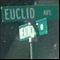Registered: 2005-12-04
Posts: 3,791

### Re: Find a number...

1 of course is the simplest non-zero answer.  In order to solve for a solution, one must solve the equation:

Solving this equation for a, we get:

The trick now is to find b such that -36b + 100 is a perfect square.  0 works, but it leads to the trivial solution (i.e. 0) and a = 10.  1 works as well, which leads to both 81 and 1 itself.

"In the real world, this would be a problem.  But in mathematics, we can just define a place where this problem doesn't exist.  So we'll go ahead and do that now..."

Offline

## #5 2008-06-08 14:45:13

JaneFairfax
MemberRegistered: 2007-02-23
Posts: 6,868

### Re: Find a number...

A faster method:

0 = 0 (yes)
1 = 1 (yes)
4 = 4 (no)
9 = 9 (no)
1 + 6 = 7 (no)
2 + 5 = 7 (no)
3 + 6 = 9 (no)
4 + 9 = 13 (no)
6 + 4 = 10 (no)
8 + 1 = 9 (yes)
1 + 0 + 0 = 1 (no)
1 + 2 + 1 = 4 (no)
.
.
.
etc etc etc

Last edited by JaneFairfax (2008-06-08 14:45:59)

Offline

## #6 2008-06-08 15:35:43

Ricky
ModeratorRegistered: 2005-12-04
Posts: 3,791

### Re: Find a number...

Jane, that method isn't based in logic, it's just an algorithmic test by exhaustion.

"In the real world, this would be a problem.  But in mathematics, we can just define a place where this problem doesn't exist.  So we'll go ahead and do that now..."

Offline

## #7 2008-06-09 00:24:24

JaneFairfax
MemberRegistered: 2007-02-23
Posts: 6,868

### Re: Find a number...

Proof by exhaustion is still a logical proof.

Offline

## #8 2008-06-09 03:18:40

Ricky
ModeratorRegistered: 2005-12-04
Posts: 3,791

### Re: Find a number...

I believe you're not interpreting his words properly.  The way I interpreted them is that "logical" meant that you didn't go by exhaustion, that you used logic (math) to find them instead.

"In the real world, this would be a problem.  But in mathematics, we can just define a place where this problem doesn't exist.  So we'll go ahead and do that now..."

Offline

## #9 2008-06-09 03:24:48

mathsyperson
Moderator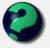Registered: 2005-06-22
Posts: 4,900

### Re: Find a number...

Would it count as logical if you proved that no number bigger than, say, 50 could possibly fit the condition, and then tested all the others individually (treating them as special cases)?

Why did the vector cross the road?
It wanted to be normal.

Offline

## #10 2008-06-09 04:18:32

Ricky
ModeratorRegistered: 2005-12-04
Posts: 3,791

### Re: Find a number...

Typically, yes.  However, this does not seem to be in the same sense that ZHero is using the word.

"In the real world, this would be a problem.  But in mathematics, we can just define a place where this problem doesn't exist.  So we'll go ahead and do that now..."

Offline

## #11 2008-06-10 09:20:54

ZHero
Real MemberRegistered: 2008-06-08
Posts: 1,889

### Re: Find a number...

Hi guys!
Firstly, i'm new to the site & i'm really pleased to see so many people interested/engrossed in Mathematics!
Maths, no doubts, is most Beautiful, Puzzling & Fathomless of all things!

I think i've found a nice Mathematical way to crack the above problem but i'll find it a bit difficult to post it coz i don't know how to code for equations n i'm surfing through my Nokia6600 rather than computer..
Here it goes...

If two or more thoughts intersect, there has to be a point!

Offline

## #12 2008-06-10 09:51:11

ZHero
Real MemberRegistered: 2008-06-08
Posts: 1,889

### Re: Find a number...

Let no. be 10x+y (x,y=0-9;x=!0).
Now..
10x + y = (x+y)^2
9x = (x+y)^2 - (x+y)
let's say x + y = z
so..
9x = z^2 - z = z(z-1)
consider rhs.. Its a product of two CONSECUTIVE numbers!
So, 9x should be multiple of two CONSECUTIVE numbers & x MUST be a ONE DIGIT number which leads to only consecutive number to 9 i.e. '8'!
So.. x = 8 is the only solution which in turn gives y = 1!
So the number is 81!

If two or more thoughts intersect, there has to be a point!

Offline

## #13 2008-06-10 10:12:35

ZHero
Real MemberRegistered: 2008-06-08
Posts: 1,889

### Re: Find a number...

The required number can only be 'one/two digit' number can also be shown..
An 'n digit' number 'a' would satisfy the above question only if "square of the sum of the digits of the GREATEST 'n digit' number EQUALS/EXCEEDS the number'!

Greatest 'one digit' number is 9..
9^2 = 81 > 9... Satisfies!

Greatest 'two digit' number is 99..
9+9 = 18
18^2 = 324 > 99... Satisfies!

Greatest 'three digit' number is 999..
9+9+9 = 27
27^2 = 729 < 999.. Doesn't Satisfy!!
& so on...

Regards...

If two or more thoughts intersect, there has to be a point!

Offline

## #14 2008-06-10 10:33:37

mathsyperson
ModeratorRegistered: 2005-06-22
Posts: 4,900

### Re: Find a number...

ZHero wrote:

The required number can only be 'one/two digit' number can also be shown..
An 'n digit' number 'a' would satisfy the above question only if "square of the sum of the digits of the GREATEST 'n digit' number EQUALS/EXCEEDS the number'!

I don't agree with this.
For example, take 199. 1+9+9 = 19 and 19² = 361 > 199, so even though the highest 3-digit number is greater than the sum of its digits, there are still some 3-digit numbers that are less (which means there's no reason why there couldn't be some that are equal).

Testing exhaustively shows that there aren't any, but you couldn't say that without trying them all.
However, you could use a similar argument to prove there aren't any 5-digit (or higher) numbers with that property though.

The highest sum-of-digits-squared number would be made by 99999.
5x9 = 45, and 45² = 2025.
The highest sum-of-digits-squared number is lower than the lowest 5-digit number (10000), and so there can't be any 5-digit numbers equal to the squared sum of their digits.

Why did the vector cross the road?
It wanted to be normal.

Offline

## #15 2008-06-10 11:49:44

JaneFairfax
MemberRegistered: 2007-02-23
Posts: 6,868

### Re: Find a number...

Well, then. It looks like what ZHero means by logically is somewhere between my proof by exhaustion and Rickys algebraic proof.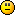Offline

## #16 2008-06-10 14:06:07

ZHero
Real MemberRegistered: 2008-06-08
Posts: 1,889

### Re: Find a number...

Very well said mathsyperson!
I'm thinking again..
There's ONLY ONE such number & hence it should be uniquely determinable!!

Consider for example..
Find a number which is square of its last digit (digit in unit's place)?
Ans (quite obvious) is 25 & 36 and these can be determined using almost the same logic as above, for solving the equation!

If two or more thoughts intersect, there has to be a point!

Offline

## #17 2010-05-27 17:41:43

ZHero
Real MemberRegistered: 2008-06-08
Posts: 1,889

### Re: Find a number...

Consider a three digit number (100a+10b+c) ; a, b, c ∈ {0, 1, 2, 3, 4, 5, 6, 7, 8, 9} and a≠0

(100a+10b+c) = (a+b+c)[sup]2[/sup]
99a+9b = (a+b+c)[sup]2[/sup] - (a+b+c)
9(11a+b) = (a+b+c)(a+b+c-1)

Since LHS of the equation is a Multiple of 9 and that the Maximum Value of RHS (a+b+c) can be (9+9+9) = 27, we have the following three cases...

CASE-I: 9(11a+b) = 9×8
11a+b = 8 a=1 and b=-3 Not Possilbe (b can't be -ve)

CASE-II: 9(11a+b) = 18×17
11a+b = 34 a=3 and b=1

a+b+c = 18
c = 18 - (a+b) = 18 - (3+1) = 14 Not Possilbe (c=14 is not possible)

CASE-III: 9(11a+b) = 27×26
11a+b = 78 a=7 and b=1
a+b+c = 27
c = 27 - (a+b) = 27 - (7+1) = 19 Not Possilbe (c=19 is not possible)

Hence, we can conclude that NO SUCH THREE DIGIT NUMBER IS POSSIBLE!

I think it'd be much easier to show this for higher digit numbers!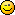If two or more thoughts intersect, there has to be a point!

Offline

## #18 2010-05-28 03:31:40

TheDude
Member
Registered: 2007-10-23
Posts: 361

### Re: Find a number...

You need to check 2 more cases.

CASE-IV: 9(11a+b) = 10x9
11a+b = 10 ⇒ a=1 and b=-1 Not Possible

CASE-V: 9(11a+b) = 19x18
11a+b = 38 ⇒ a=3 and b=5
a+b+c = 19
c = 19 - (a+b) = 19 - (3+5) = 11 Not Possible

Wrap it in bacon

Offline

## #19 2010-05-28 16:47:52

ZHero
Real MemberRegistered: 2008-06-08
Posts: 1,889

### Re: Find a number...

TheDude wrote:

You need to check 2 more cases.

CASE-IV: 9(11a+b) = 10x9
11a+b = 10 ⇒ a=1 and b=-1 Not Possible

CASE-V: 9(11a+b) = 19x18
11a+b = 38 ⇒ a=3 and b=5
a+b+c = 19
c = 19 - (a+b) = 19 - (3+5) = 11 Not Possible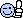I always miss one thing or the other..........If two or more thoughts intersect, there has to be a point!

Offline